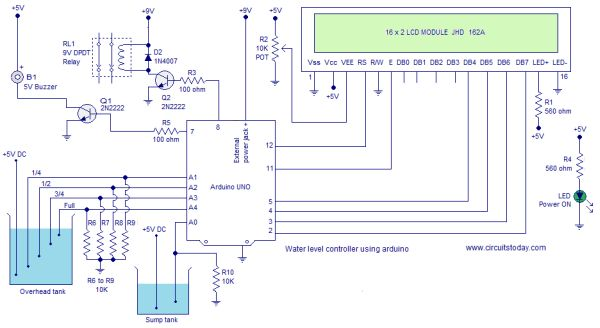# Water level controller using arduino

### Arduino water level controller / indicator.

This article is a about a fully functional water level controller using Arduino. The circuit displays the  level of water in the tank and switches the motor ON when the water level goes below  a predetermined level. The circuit automatically switches the motor OFF when the tank is full. The water level and other important data are displayed on a 16×2 LCD display. The circuit also monitors the level of water in the sump tank (source tank). If the level in side the sump tank is low, the motor will not be switched ON and this protects the motor from dry running. A beep sound is generated when the level in the sump tank is low or if there is any fault with the sensors.

#### Circuit diagram.The circuit diagram of the water level controller using Arduino is shown above. Conductive method is used to measure the level. The sensor assembly consists of four aluminum wires arranged at 1/4, 1/2, 3/4 and full levels in the tank. The dry ends of these wires are connected to analog input pins A1, A2, A3 and A4 of the Arduino respectively. A  fifth wire is positioned at the bottom of the tank. Resistors R6 to R9 are pull down resistors.The dry end of this wire is connected to +5V DC. When the water touches a particular probe, electrical connection is established between that probe and the +5V probe because water has slight conductivity. As a result current flows through that probe and this current is converted into a proportional voltage by the pull down resistor. Arduino reads the voltage dropped across each pull down resistor for sensing the level of water in the tank. Same method is used for measuring the level of water in the sump tank.

For more detail: Water level controller using arduino

#### This Post / Project can also be found using search terms:

• water level controller using arduino
• water level control arduino
• arduino used in water level control
• waterlevel project using arduino